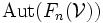# Automorphism group of finitely generated free group

## Definition

Let$n$ be a natural number. The automorphism group of the free group on$n$ generators is defined as the automorphism group of$F_n$, the free group on$n$ generators. A group is termed the automorphism group of a finitely generated free group if it is isomorphic to$\operatorname{Aut}(F_n)$ for some natural number$n$.

## Relation with other groups

### General relation with reduced free groups

Let$\mathcal{V}$ be any subvariety of the variety of groups. If$F_n(\mathcal{V})$ denotes the free algebra on$n$ generators in$\mathcal{V}$, then$F_n(\mathcal{V})$ is the quotient of$F_n$ by a verbal subgroup. Groups of the form$F_n(\mathcal{V})$ are termed reduced free groups.

There is a natural homomorphism from$\operatorname{Aut}(F_n)$ to$\operatorname{Aut}(F_n(\mathcal{V}))$, which sends an automorphism of the free group to the induced automorphism of$F_n(\mathcal{V})$. One way of seeing this is to observe that$F_n(\mathcal{V})$ is the quotient of$F_n$ by a verbal subgroup, which is in particular a characteristic subgroup, hence any automorphism of$F_n$ descends to an automorphism of the quotient.

However, this homomorphism is not necessarily surjective. For instance, if$\mathcal{V}$ is given as the variety of abelian groups where every element has order$p$ for some prime$p \ge 5$, there are automorphisms of$F_1(\mathcal{V} = \mathbb{Z}/p\mathbb{Z}$ that do not arise from automorphisms of$F_1 = \mathbb{Z}$.

### Relation with free abelian groups

Free abelian groups are free algebras in the variety of abelian groups. The free abelian group of rank$n$ is isomorphic to$\mathbb{Z}^n$, and its automorphism group is isomorphic to$GL(n,\mathbb{Z})$. Thus, we have a homomorphism:$\operatorname{Aut}(F_n) \to GL(n,\mathbb{Z})$.

It turns out that this automorphism is surjective -- in other words, every automorphism of the free abelian group on$n$ generators arises from an automorphism of the free group on$n$ generators.

## IAPS structure

Further information: IAPS of automorphism groups of free groups

The collection of groups$\operatorname{Aut}(F_n)$ form an IAPS of groups. In other words, we can construct injective homomorphisms:$\Phi_{m,n}: \operatorname{Aut}(F_m) \times \operatorname{Aut}(F_n) \to \operatorname{Aut}(F_{m+n})$

satisfying the associativity condition.